# Hard sphere modelPhase diagram (pressure vs packing fraction) of hard sphere system (Solid line - stable branch, dashed line - metastable branch)

The hard sphere model (sometimes known as the rigid sphere model) is defined as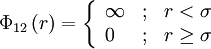$\Phi_{12}\left( r \right) = \left\{ \begin{array}{lll} \infty & ; & r < \sigma \\ 0 & ; & r \ge \sigma \end{array} \right.$

where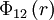$\Phi_{12}\left(r \right)$ is the intermolecular pair potential between two spheres at a distance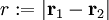$r := |\mathbf{r}_1 - \mathbf{r}_2|$, and$\sigma$ is the diameter of the sphere. The hard sphere model can be considered to be a special case of the hard ellipsoid model, where each of the semi-axes has the same length,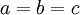$a=b=c$.

## First simulations of hard spheres (1954-1957)

The hard sphere model, along with its two-dimensional manifestation hard disks, was one of the first ever systems studied using computer simulation techniques with a view to understanding the thermodynamics of the liquid and solid phases and their corresponding phase transition   , much of this work undertaken at the Los Alamos Scientific Laboratory on the world's first electronic digital computer ENIAC .

## Liquid phase radial distribution function

The following are a series of plots of the hard sphere radial distribution function  shown for different values of the number density$\rho$. The horizontal axis is in units of$\sigma$ where$\sigma$ is set to be 1. Click on image of interest to see a larger view.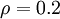$\rho=0.2$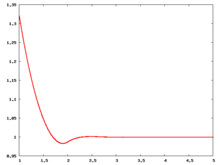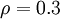$\rho=0.3$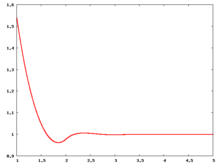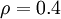$\rho=0.4$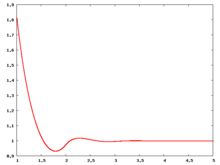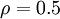$\rho=0.5$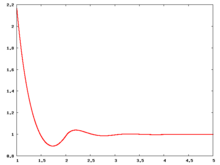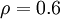$\rho=0.6$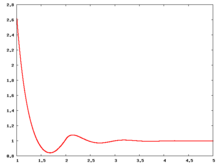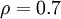$\rho=0.7$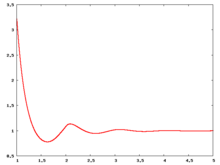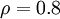$\rho=0.8$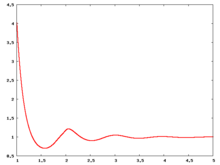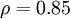$\rho=0.85$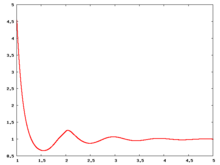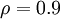$\rho=0.9$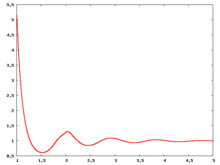The value of the radial distribution at contact,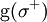${\mathrm g}(\sigma^+)$, can be used to calculate the pressure via the equation of state (Eq. 1 in )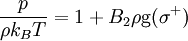$\frac{p}{\rho k_BT}= 1 + B_2 \rho {\mathrm g}(\sigma^+)$

where the second virial coefficient,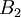$B_2$, is given by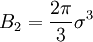$B_2 = \frac{2\pi}{3}\sigma^3$.

Carnahan and Starling  provided the following expression for${\mathrm g}(\sigma^+)$ (Eq. 3 in )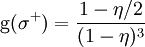${\mathrm g}(\sigma^+)= \frac{1-\eta/2}{(1-\eta)^3}$

where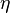$\eta$ is the packing fraction.

Over the years many groups have studied the radial distribution function of the hard sphere model:           

## Liquid-solid transition

The hard sphere system undergoes a liquid-solid first order transition  , sometimes referred to as the Kirkwood-Alder transition . The liquid-solid coexistence densities (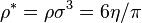$\rho^* = \rho \sigma^3=6\eta/\pi$) has been calculated to be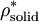$\rho^*_{\mathrm {solid}}$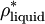$\rho^*_{\mathrm {liquid}}$ Reference 1.041(4) 0.943(4)  1.0376 0.9391  1.0367(10) 0.9387(10)  1.0372 0.9387  1.0369(33) 0.9375(14)  1.037 0.938  1.033(3) 0.935(2)  1.03715(9) 0.93890(7) 

The coexistence pressure has been calculated to be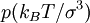$p (k_BT/\sigma^3)$ Reference 11.5727(10)  11.57(10)  11.567  11.55(11)  11.54(4)  11.50(9)  11.48(11)  11.43(17)  11.550(4) 

The coexistence chemical potential has been calculated to be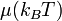$\mu (k_BT)$ Reference 15.980(11)  16.053(4) 

The Helmholtz energy function (in units of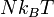$Nk_BT$) is given by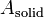$A_{\mathrm {solid}}$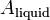$A_{\mathrm {liquid}}$ Reference 4.887(3) 3.719(8) 

The melting and crystallization process has been studied by Isobe and Krauth .

## Helmholtz energy function

Values for the Helmholtz energy function ($A$) are given in the following Table: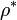$\rho^*$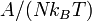$A/(Nk_BT)$ Reference 0.25 −1.766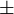$\pm$ 0.002 Table I  0.50 −0.152$\pm$ 0.002 Table I  0.75 1.721$\pm$ 0.002 Table I  1.04086 4.959 Table VI  1.099975 5.631 Table VI  1.150000 6.274 Table VI 

In  the free energies are given without the ideal gas contribution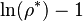$\ln(\rho^*)-1$ . Hence, it was added to the free energies in the table.

## Interfacial Helmholtz energy function

The Helmholtz energy function of the solid–liquid interface has been calculated using the cleaving method giving (Ref.  Table I):

 work per unit area/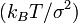$(k_BT/\sigma^2)$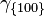$\gamma_{\{100\}}$ 0.5820(19)$\gamma_{\{100\}}$ 0.636(11)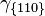$\gamma_{\{110\}}$ 0.5590(20)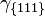$\gamma_{\{111\}}$ 0.5416(31)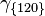$\gamma_{\{120\}}$ 0.5669(20)

## Solid structure

The Kepler conjecture states that the optimal packing for three dimensional spheres is either cubic or hexagonal close packing, both of which have maximum densities of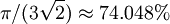$\pi/(3 \sqrt{2}) \approx 74.048%$  . However, for hard spheres at close packing the face centred cubic phase is the more stable , with a Helmholtz energy function difference in the thermodynamic limit between the hexagonal close packed and face centered cubic crystals at close packing of 0.001164(8)$Nk_BT$. Recently evidence has been found for a metastable cI16 phase  indicating the "cI16 is a mechanically stable structure that can spontaneously emerge from a bcc starting point but it is thermodynamically metastable relative to fcc or hcp".

## Direct correlation function

For the direct correlation function see:  

## Bridge function

Details of the bridge function for hard sphere can be found in the following publication 

## Equations of state

Main article: Equations of state for hard spheres

## Virial coefficients

Main article: Hard sphere: virial coefficients

## Experimental results

Pusey and van Megen used a suspension of PMMA particles of radius 305$\pm$10 nm, suspended in poly-12-hydroxystearic acid  For results obtained from the Colloidal Disorder - Order Transition (CDOT) experiments performed on-board the Space Shuttles Columbia and Discovery see Ref. 

## Related systems

Hard spheres in other dimensions: